# Clausius- Mosotti relation between polarizability and dielectric constant

The Clausius-Mosotti relation is an equation that relates the dielectric constant (ε) of a polar dielectric material to its molecular properties. It provides an approximate relationship between the dielectric constant and the polarizability of the molecules within the material.

This relation connects the dielectric constant of insulator to atomic polarizability. The local electric field acting on an atom in a general lattice site of dielectric can be written asThe sum of applied field and depolarization field is known as Macroscopic electric field i.eLet's calculate the Lorentz field. Let us consider fictitious spherical cavity within the dielectric as shown in figure. If θ is the polar angle referred to polarization direction the surface charge density on the surface of the cavity is -pcosθ. The electrical field at the centre of the spherical cavity of radius 'a' is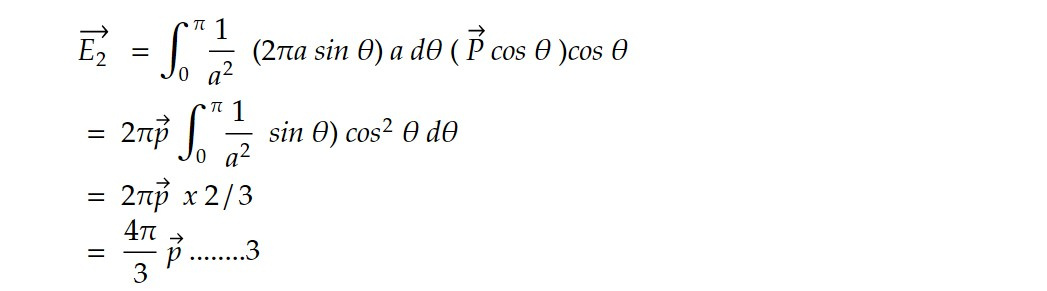For the atom at the cubic site of a dielectric vector E3 = 0 .........4

Thus from 1,2,3 and 4, we can write local field action on an atom in a cubic site of dielectric asThe dipole moment of a single atom is proportional to local electrical field as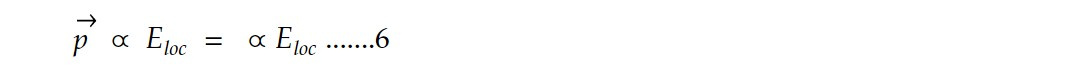The polarisation is given by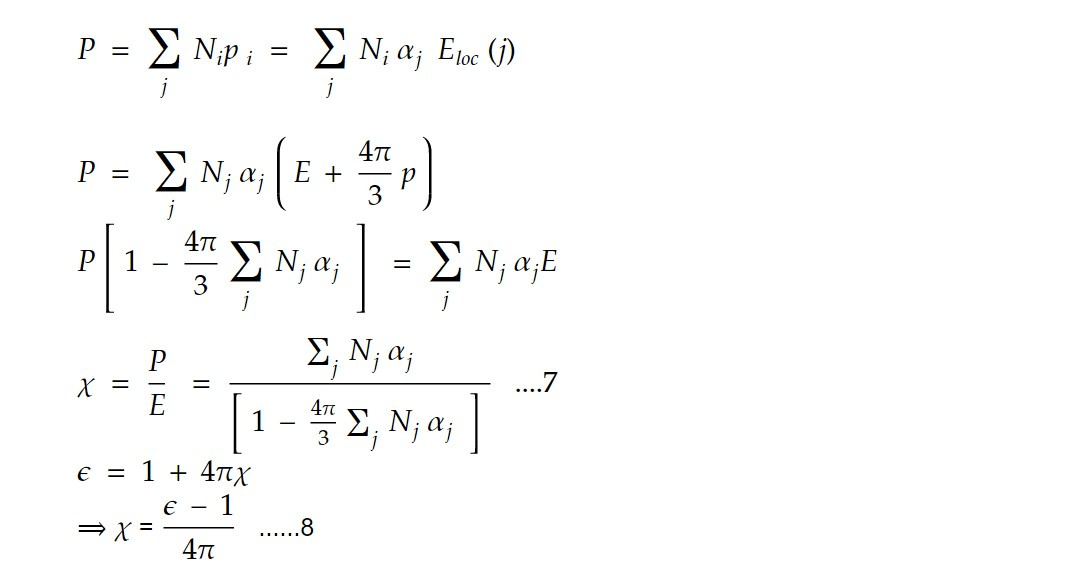Thus from 7 and 8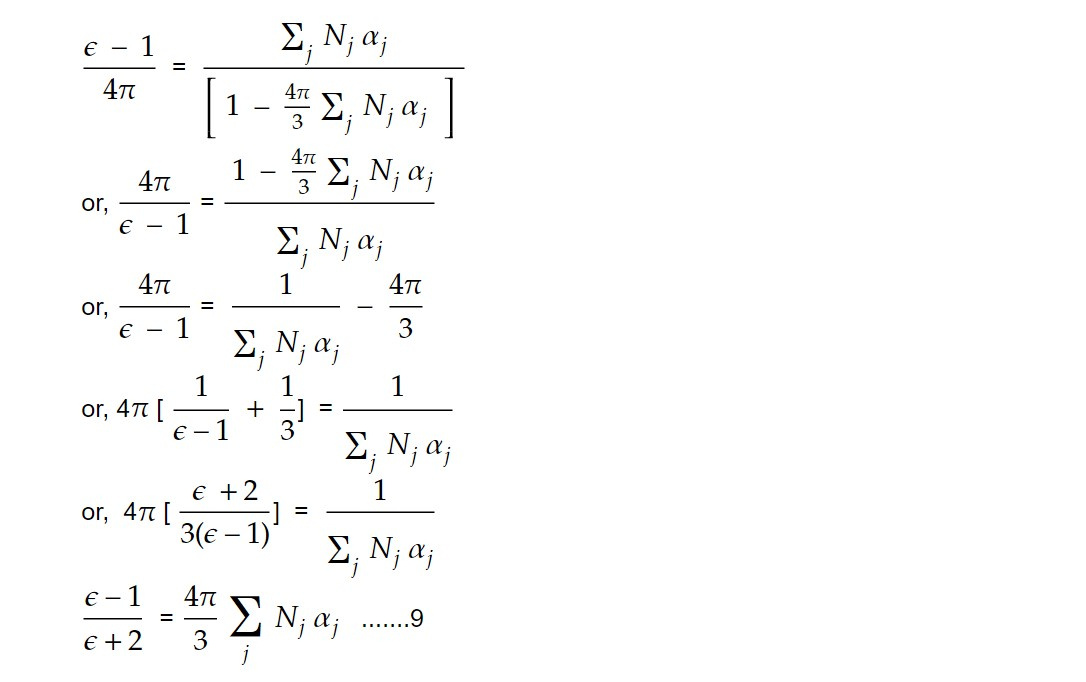For polar dielectrics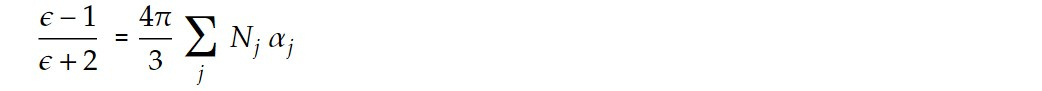The Clausius- Mosotti relation can be written as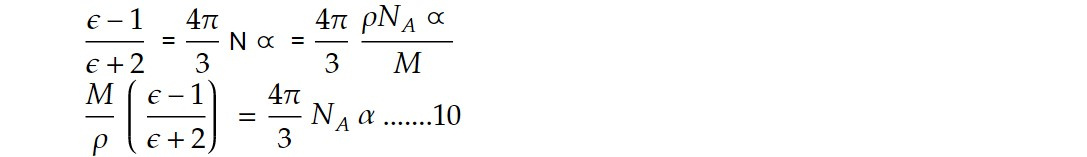In this equation, N represents the number density of polarizable molecules in the material, and α is the average polarizability of the molecules.

The dielectric constant (ε) measures the ability of a material to store electrical energy when subjected to an electric field. It is a dimensionless quantity that describes the extent to which the electric field is weakened or enhanced within the material compared to the vacuum.

Now the Clausius- Mosotti relation for the polar dielectrics can be written as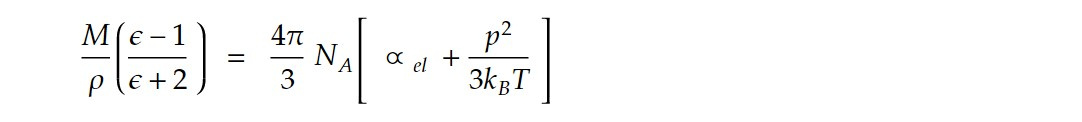The Clausius-Mosotti relation provides an estimation of the dielectric constant based on the polarizability of the constituent molecules. It assumes that the polarizable molecules behave as independent dipoles in response to an electric field.

It's important to note that the Clausius-Mosotti relation is an approximation and does not account for all factors that influence the dielectric properties of a material. Real-world dielectric behavior is often more complex, and additional factors such as intermolecular interactions and the presence of defects or impurities can affect the dielectric constant. Nonetheless, the Clausius-Mosotti relation provides a useful framework for understanding the relationship between dielectric properties and molecular polarizability in polar dielectrics.

This note is a part of the Physics Repository.